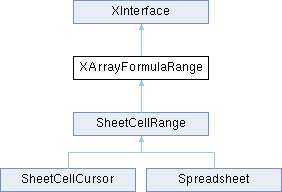LibreOffice LibreOffice 7.4 SDK API Reference
XArrayFormulaRange Interface Referencepublished

provides handling of array formulas in a cell range. More...

`import"XArrayFormulaRange.idl";`

Inheritance diagram for XArrayFormulaRange:## Public Member Functions

string getArrayFormula ()
returns the array formula of the range or an empty string, if the range does not contain an array formula. More...

void setArrayFormula ([in] string aFormula)
applies the array formula to the entire cell range. More...Public Member Functions inherited from XInterface
any queryInterface ([in] type aType)
queries for a new interface to an existing UNO object. More...

void acquire ()
increases the reference counter by one. More...

void release ()
decreases the reference counter by one. More...

## Detailed Description

provides handling of array formulas in a cell range.

Developers Guide
Spreadsheet Documents - Handling Array Formulas

## ◆ getArrayFormula()

 string getArrayFormula ( )

returns the array formula of the range or an empty string, if the range does not contain an array formula.

## ◆ setArrayFormula()

 void setArrayFormula ( [in] string aFormula )

applies the array formula to the entire cell range.

Parameters
 aFormula the formula that will be applied as array formula. Passing an empty string erases an existing array formula.

The documentation for this interface was generated from the following file: